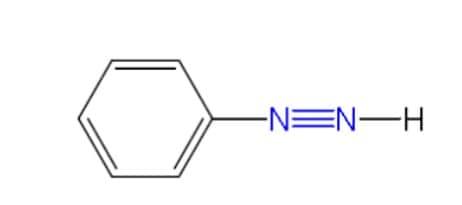# NMAT Chemistry Practice Test

Last Updated on 12/21/2019 by FilipiKnowDirections: Choose the letter that corresponds to the correct answer.

1. The chart below shows the acid-base testing of four samples.Based on the chart, which sample or samples are acids?

A. Sample 1 only

B. Samples 2 and 4

C. Samples 1 and 3

D. Sample 4 only

2. The graph below represents the phase changes of water.Based on the graph, what change occurred when ice at 0 degrees Celsius was heated at a constant rate?

A. Molecules broke apart into atoms.

B. Elements dissociated into ions.

C. Intermolecular attractions were decreased.

D. Chemical energy was stored.

3. When the volume of a gas is decreased at a constant temperature, the pressure increases because the molecules

A. move faster

B. move slower.

C. become heavier.

D. become lighter.

E. strike a unit area of the container more often.

4. Which property do the liquid phase and the gas phase of a substance have in common?

A. Both phases are highly compressible.

B. Both phases lack a definite shape.

C. Both phases have high densities.

D. Both phases have equal kinetic energy.

5. What volume of 12.0 M HCl is required to make 75.0 mL of 3.50 M HCl?

A. 21.9 mL

B. 0.560 mL

C. 257 mL

D. 75.0 mL

6. Air pollution from automobile exhaust is minimized by using electric cars powered by lead-acid batteries. What will be a negative effect of using lead-acid batteries?

A. Toxic metal in the batteries will enter the environment.

B. Sulfuric acid in the batteries will generate electricity.

C. Oxygen and hydrogen will be produced by the batteries.

D. Dense and malleable metal will be used in the batteries.

7. Which set of tools will provide the most precise measurements for calculating the density of an irregularly shaped rock?

8. Water has a higher boiling point than compounds of similar molecular weight. Which of the following best explains this phenomenon?

A. Extensive hydrogen bonding exists between water molecules.

B. One of the natural isotopes of hydrogen, deuterium, is present in sufficient quantities to significantly raise the boiling point.

C. Water is a polar covalent compound.

D. Van der Waals forces exist between individual water molecules.

E. Water is largely dissociated leading to large electrostatic forces between individual water molecules.

9. According to IUPAC nomenclature, which of the following is the name for the compound shown below?A. 3-butyl-2-(l-methylethyl)pentane

B. 2-isopropyl-3-butylpentane

C. 2-isopropyl-3-ethylheptane

D. 4-ethyl-2,3-dimethyloctane

E. 2-isododecane

10. What is the molarity of a 2.0-liter solution containing 58 grams of NaCl?

A. 0.50 M

B. 1.0 M

C. 2.0 M

D. 3.2 M

11. Which statement best describes the Bohr model of the atom?

A. Electrons are arranged in energy clouds.

B. Electrons have properties similar to waves.

C. Electrons orbit around the nucleus in set paths.

D. Electrons make up most of the mass of an atom.

12. The statements below explain why magnesium is preferred over zinc to protect underground iron pipes in terms of reactivity EXCEPT for:

A. Magnesium is more active than zinc

B. Magnesium atoms lose electrons more easily than zinc atoms

C. Zinc is more active than magnesium

D. Magnesium oxidized more readily than zinc

13. The electronic configuration of a particular neutral atom is 1s²2s²2p63s²3p². What is the number of unpaired electrons in this atom?

A. 1

B. 2

C. 3
D. 4

E. 0

14. What volume will 50.2 grams of CO2(g) occupy at STP?

A. 1.14 liters

B. 19.6 liters

C. 25.6 liters

D. 98.2 liters

15. All of the following statements about carbon dioxide are true EXCEPT:

A. It can be prepared by the action of acid on limestone.

B. It is used to extinguish fires.

C. It dissolves in water at room temperature.

D. It sublimes rather than melts at 20 degrees Celsius and 1-atmosphere pressure.

E. It is less dense than air at a given temperature and pressure.

16. A gas has a volume of 3.0 L at a pressure of 3.0 atm. What will be the final volume of the gas if the pressure is increased to 5.0 atm at a constant temperature?

A. 0.56 L

B. 1.8 L

C. 3.0 L

D. 5.0 L

17. The bulb of the open-end manometer shown below contains a gas.I. Only atmospheric pressure is exerted on the exposed mercury surface on the right side of the tube.

II. The gas pressure is greater than atmospheric pressure.

III. The difference in the height, h, of mercury levels is equal to the pressure of the gas.

A. II only

B. III only

C. I and II only

D. I and III only

E. I, II, and III

18. What is the total number of electrons shared in the bonds between the two nitrogen atoms in the following molecule?A. 2

B. 8

C. 6

D. 3

19. What is the molarity of a 0.5 L sample of solution that contains 60.0 g of sodium hydroxide (NaOH)?

A. 0.8 M

B. 1.5 M

C. 3.0 M

D. 6.0 M

20. Of the following materials, which contribute(s) most to the production of acid rain?

A. Uranium hexafluoride

B. Ozone

C. Phosphate detergents

D. Nitric oxide

E. Chlorofluorocarbons

View answer keyor go back to NMAT reviewer main pageFilipiKnow

FilipiKnow strives to ensure each article published on this website is as accurate and reliable as possible. We invite you, our reader, to take part in our mission to provide free, high-quality information for every Juan. If you think this article needs improvement, or if you have suggestions on how we can better achieve our goals, let us know by sending a message to admin at filipiknow dot net

## 2 thoughts on “NMAT Chemistry Practice Test”

1. Pingback: NMAT Chemistry Answer Key
2. Pingback: NMAT Chemistry Answer Key - FilipiKnow

This site uses Akismet to reduce spam. Learn how your comment data is processed.